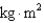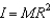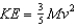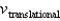Energy and Momentum of Rotational Motion

# College Physics Reasoning and Relationships

Physics & Astronomy

## Quiz 9 :Energy and Momentum of Rotational MotionStudy FlashcardsLooking for Introductory Physics Homework Help?## Quiz 9 :Energy and Momentum of Rotational Motion

Showing 1 - 20 of 52Two spheres, one with the center core up to r = R/2 hollow and the other solid, have the same mass M and same outer radius R. If they are both rolling at the same linear speed, which one has the greater kinetic energy?
Free
Multiple Choice

BA ventilation fan with a moment of inertia of 0.034has a net torque of 0.11 Nm applied to it. If it starts from rest, what kinetic energy will it have 10 s later?
Free
Multiple Choice

BThe total kinetic energy of a baseball thrown with a spinning motion is a function of:
Free
Multiple Choice

CA bowling ball has a mass of 7.0 kg, a moment of inertia of 2.8 102, and a radius of 0.10 m. If it rolls down the lane without slipping at a linear speed of 3.0 m/s, what is its total kinetic energy?
Multiple ChoiceA bucket of water with total mass 23 kg is attached to a rope, which in turn is wound around a 0.050-m radius cylinder, with crank, at the top of a well. The moment of inertia of the cylinder and crank is 0.21 kgm2. The bucket is raised to the top of the well and released to fall back into the well. What is the kinetic energy of the cylinder and crank at the instant the bucket is moving with a speed of 6.0 m/s?
Multiple ChoiceA gyroscope has a moment of inertia of 0.14and an initial angular speed of 30 rad/s. Friction in the bearings causes its speed to reduce to zero in 30 s. What is the value of the average frictional torque?
Multiple ChoiceA gyroscope has a moment of inertia of 0.140 kgm2 and has an initial angular speed of 12.0 rad/s. If a lubricant is applied to the bearings of the gyroscope so that frictional torque is reduced to 2.00 102 NM, then in what time interval will the gyroscope coast from 12.0 rad/s to zero?
Multiple ChoiceA cylinder with its mass concentrated toward the center has a moment of inertia of 0.2MR2. If this cylinder is rolling without slipping along a level surface with a linear speed v, what is the ratio of its rotational kinetic energy to its linear kinetic energy?
Multiple ChoiceA hoop with mass, M, and radius, R, rolls along a level surface without slipping with a linear speed, v. What is the ratio of rotational to linear kinetic energy? (For a hoop,.)
Multiple ChoiceA rotating flywheel can be used as a method to store energy. If it is required that such a device be able to store up to a maximum of 2.00 106 J when rotating at 400 rad/s, what moment of inertia is required?
Multiple ChoiceA rotating flywheel can be used as a method to store energy. If it has 2.0 106 J of kinetic energy when rotating at 400 rad/s, and if a frictional torque of 4.0 Nm acts on the system, in what interval of time would the flywheel come to rest?
Multiple ChoiceAn initially installed flywheel can store 106 J of kinetic energy when rotating at 300 rad/s. It is replaced by another flywheel of the same size but made of a stronger material. If its mass is the same as that of the original, and it is now capable of achieving a rotational speed of 600 rad/s, what maximum energy can be stored?
Multiple ChoiceA bus is designed to draw its power from a rotating flywheel that is brought up to its maximum speed (3000 rpm) by an electric motor. The flywheel is a solid cylinder of mass 250 kg and radius 0.500 m (Icylinder = MR2/2). If the bus requires an average power of 10.0 kW, how long will the flywheel rotate?
Multiple ChoiceA solid sphere, a solid cylinder, and a hoop each have the same mass and radius. If they are spinning at the same angular velocity, which one has the greatest rotational kinetic energy?
Multiple ChoiceThe Earth's density increases with depth. If instead the Earth had uniform density, but the same angular velocity and mass, how would its rotational kinetic energy compare to the actual value?
Multiple ChoiceA non-uniform round object of mass M and radius R is found to have its total kinetic energy to be given bywhen rolling down an incline. What is this object's moment of inertia?
Multiple ChoiceA hoop, solid sphere, and solid cylinder each have the same mass and same radius.When rolling down the same incline, which has the greatest torque from gravity on it?
Multiple ChoiceThree disks have the same mass and the same total kinetic energy. Disk #1 has radius R, disk #2 has radius 2R, and disk #3 has radius 3R. Which one has the highest?
Multiple Choice